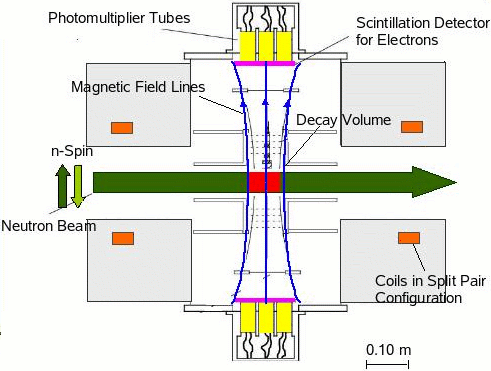# The Spectrometer Perkeo II

Home  |   Neutron Decay  |   Perkeo III  |   Perkeo II  |   The Team  |   Publications  |   Links

### The Experimental Environment

We performed the experiments (A, B) with Perkeo II at the Institut Laue-Langevin (ILL) in Grenoble, France. The beam position PF1B provides the most intense beam of cold neutrons in the world. Using the crossed geometry technique to polarize neutrons, we are able to achieve a neutron polarization of 99.7 % (preliminary).

### The Electron Spectrometer

The principle of the electron spectrometer Perkeo II is fairly simple (see figure): A beam of polarized neutrons passes through the spectrometer and some of the particles (1 in 105) decay in a certain volume. It is centered within a strong magnetic field (maximal 1 T), which is produced by two superconducting coils that are close to split pair configuration.This magnetic field separates the decay volume into two hemispheres and guides the charged particles produced in the decay onto two opposing detectors without affecting the neutron beam. Hence Perkeo II is a real 2 x 2 Pi detector where no difficult solid angle corrections have to be applied. Using the count rates of the two detectors, we can determine the experimental asymmetries Aexp and Bexp.### Measurement of the Beta Asymmetry Correlation Coefficient A

We completed data acquisition for a new measurement of the electron asymmetry A in june 2004. Precision of this measurement was improved considerably with respect to our last measurement in 1999:

• the neutron polarization was 99.7 % with a precision of 0.1 % (preliminary)
• the spinflip efficiency was 100 % (preliminary)
• the background was reduced considerably and precisely monitored
• the detector system was optimized
• statistics were improved

Some photos of the 2004 beam time at the ILL in Grenoble (France) can be found here.

### Measurement of the Neutrino and Proton Asymmetry Correlation Coefficients B and C

With the project Perkeo IIB we measured another neutron decay correlation coefficient: The neutrino- asymmetry B. This is the correlation between neutron spin and neutrino momentum.

Largely, we used the setup of the 2003/2004 A-measurement again. We only had to narrow the beam width to minimize the magnetic mirror effect, and we had to use a different detector system (see below) to measure B. The experiment was performed from june to august 2004 at the ILL (Grenoble).

The results of the measurement are the neutrino asymmetry parameter B = 0.9802(50) [Phys. Rev. Lett. 99, 191803 (2007); arXiv:0706.3788], and the proton asymmetry parameter C = -0.2377(26) [Phys. Rev. Lett. 100, 151801 (2008), arXiv:0712.2442].

#### Physical Motivation

In the Standard Model of Particle Physics parity is maximally violated, but there is no explanation for this symmetry breaking within the model. There are theories beyond the standard model that propose full parity conservation in the early universe (left-right-symmetric models). If these models are correct it should be possible to observe weak right-handed currents. The neutrino-asymmetry B is very sensitive to these currents.

#### The Spectrometer

The electron spectrometer Perkeo II consists out of two superconducting coils in almost split pair configuration (see figure). The coils produce a 1 Tesla magnetic field perpendicular to the neutron beam. Since the charged decay products (electrons, protons) propagate along the field lines, the spectrometer is divided into two hemispheres. A detector is placed at each side.#### The Detector

Since neutrinos cannot be detected directly, we observe electron and proton from the beta decay and determine the neutrino momentum using momentum conservation. Due to the magnetic field there are two possibilities: Electron and proton can be detected in the same or in opposite hemispheres. Hence it must be possible to detect electrons and protons with the same detector. We developed a special detector setup for this problem: The protons are accelerated towards a thin carbon-foil on high voltage (-18 kV), where they are converted into electrons. These are detected by the same plastic scintillators that detect the primary electrons out of the decay.

Webmaster:
EDV Abteilung